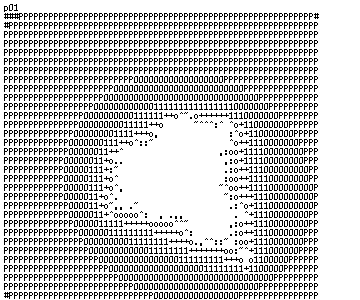# MANDELBROT ROTOZOOM

188 wordsMathieu 'p01' Henri on September 1st, 2006

# Mandelbrot rotozoom

Many people did fractals renderers in Javasript in 256 bytes, but no one ever made one that zooms and rotate... until today.## Source code

``<pre id=B><script>setInterval("for(d='p01?*?:,~.????',C=Math.cos(n-=88),S=Math.sin(n),Y=m=1+C,z=2048;z;Y-=m/16)for(d+='<br>',X=m;--z&63;X-=m/32,d+=d.charAt(k))for(r=i=k=0;++k+r*r+i*i<13;i=t)t=2*r*i-X*C+Y*S,r=r*r-i*i-X*S-Y*C+C-1;B.innerHTML=d",n=9)</script>``

Exactly 256 bytes.

And here is a slightly beautified version of the render loop

``````for(d='p01?*?:,~.????',C=Math.cos(n-=88),S=Math.sin(n),Y=m=1+C,z=2048;z;Y-=m/16)
for(d+='<br>',X=m;--z&63;X-=m/32,d+=d.charAt(k))
for(r=i=k=0;++k+r*r+i*i<13;i=t)
t=2*r*i-X*C+Y*S,
r=r*r-i*i-X*S-Y*C+C-1;
B.innerHTML=d``````

Not much magic going here. It is rather straight forward implementation of a Mandelbrot fractal renderer with zoom and rotation of the viewport.

Many bytes were wasted to make sure this effect works cross browser.

The one trick I like here and reused many times was to have one line with some kind credits that also serves as a look up table to render the frames.

## Feedback

You can find mandelbrot rotozoom on Pouet.net were it was well received after all. However it was my first tiny prod in JavaScript since the 256b.htm contest, and I had a hard time to please every one. On the other hand I learnt some valuable lessons.